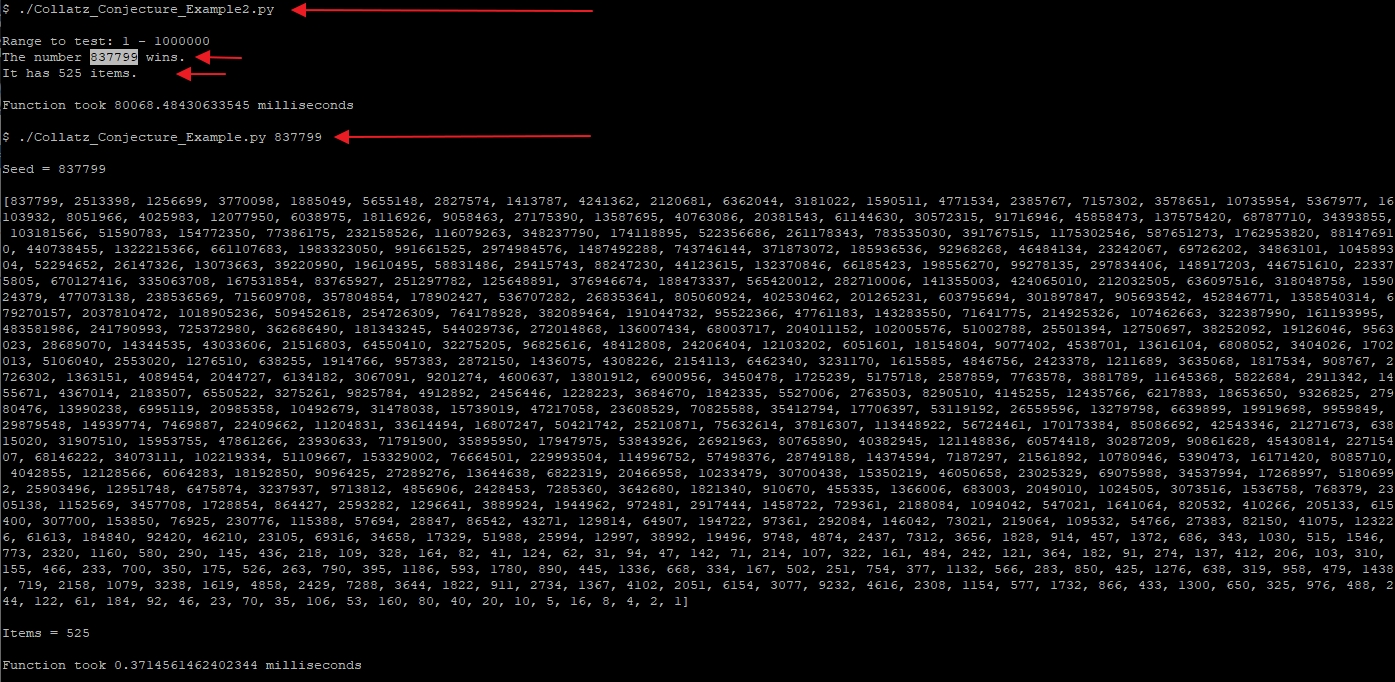Instantly share code, notes, and snippets.

# bmatthewshea/Collatz_Conjecture_Example.py

Last active August 17, 2021 18:42
Collatz Conjecture (Python Example)
This file contains bidirectional Unicode text that may be interpreted or compiled differently than what appears below. To review, open the file in an editor that reveals hidden Unicode characters. Learn more about bidirectional Unicode characters
 #!/usr/bin/python # # Collatz Conjecture: # x → x/2 (if x is even) # x → 3x + 1 (if x is odd) import sys, time # Default seed if no arguments given seed = 27 def collatz_sequence(x): seq = [x] while seq[-1] > 1: if x % 2 == 0: seq.append(int(x/2)) else: seq.append(int(3*x+1)) x = seq[-1] return seq # test for cli arguments try: if len(sys.argv)>1 : seed = int(sys.argv) except ValueError: print("Not a number.") sys.exit(1) # time the method start = time.time() # execute function list = collatz_sequence(seed) # stop/record our stopwatch finish = (time.time() - start) # print list and total list items print("\nSeed = " + str(seed) + "\n") print(list) print("\nItems = " + str(len(list))) # time spent print("\nFunction took {} milliseconds".format(1000 * finish)) #print("Script execution took {} milliseconds\n".format(1000 * (time.time() - start))) # clean exit print() sys.exit(0)
This file contains bidirectional Unicode text that may be interpreted or compiled differently than what appears below. To review, open the file in an editor that reveals hidden Unicode characters. Learn more about bidirectional Unicode characters
 # Collatz Conjecture: # x → x/2 (if x is even) # x → 3x + 1 (if x is odd) # # Calculate max amount of elements in numbers 1-(iterations): import sys, time, operator # Range to cover 1 - (iteratons - 1) iterations = 1000001 def collatz_sequence(x): seq = [x] while seq[-1] > 1: if x % 2 == 0: seq.append(int(x/2)) else: seq.append(int(3*x+1)) x = seq[-1] return seq start = time.time() # set our dictionary and for loop Fullcount = {} for number in range(1, iterations): list = collatz_sequence(number) Fullcount[number] = len(list) # print("Seed = " + str(number) + " , " + "Items = " + str(len(list))) # stop/record our stopwatch finish = (time.time() - start) # alternative way to find max key #max(Fullcount.keys(), key=(lambda k: Fullcount[k])) # find max key maximum = max(Fullcount, key=Fullcount.get) print() print("Range to test: 1 - " + str(iterations - 1) + "\n" + "The number " + str(maximum) + " wins.\nIt has " + str(Fullcount[maximum]) + " items.") # time spent print("\nFunction took {} milliseconds".format(1000 * finish)) # clean exit print() sys.exit(0)

### bmatthewshea commented Aug 17, 2021 • edited

Find max in range (Example 2 / second script) then you can show elements by running first script on same number found: